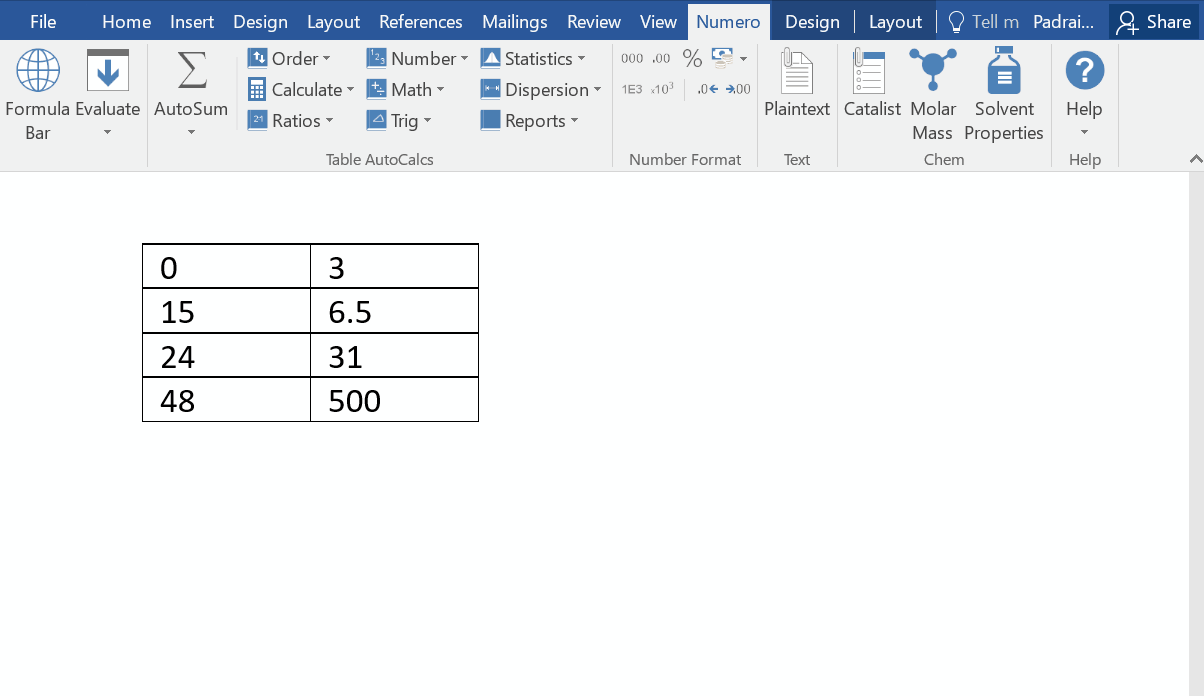Scale-up Suite Help
Table AutoCalcs
What are Table AutoCalcs?

When you have a table in Word, PowerPoint or Outlook with numbers, you can select the table and carry out some calculations on it such as Sum, Count, Standard deviation or Variance.  This section of Numero is called Table AutoCalcs, and can found on the left hand side of the Numero tab. This is shown below: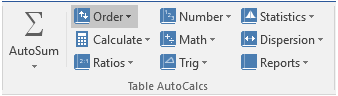AutoSum

Autosum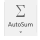can be found in the Numero ribbon in Word, PowerPoint or Outlook in the Table Autocalcs section.  Its primary use is to calculate the sum of all the selected cells in a table and copy the result onto the clipboard. The accompanied dropdown menu below the icon has additional functions such as Average Count the cells that contain number and finding the minimum and maximum of range of numbers in a table.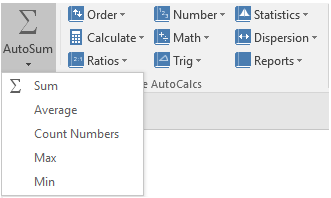For example:

1. Calculate the weekly total using AutoSum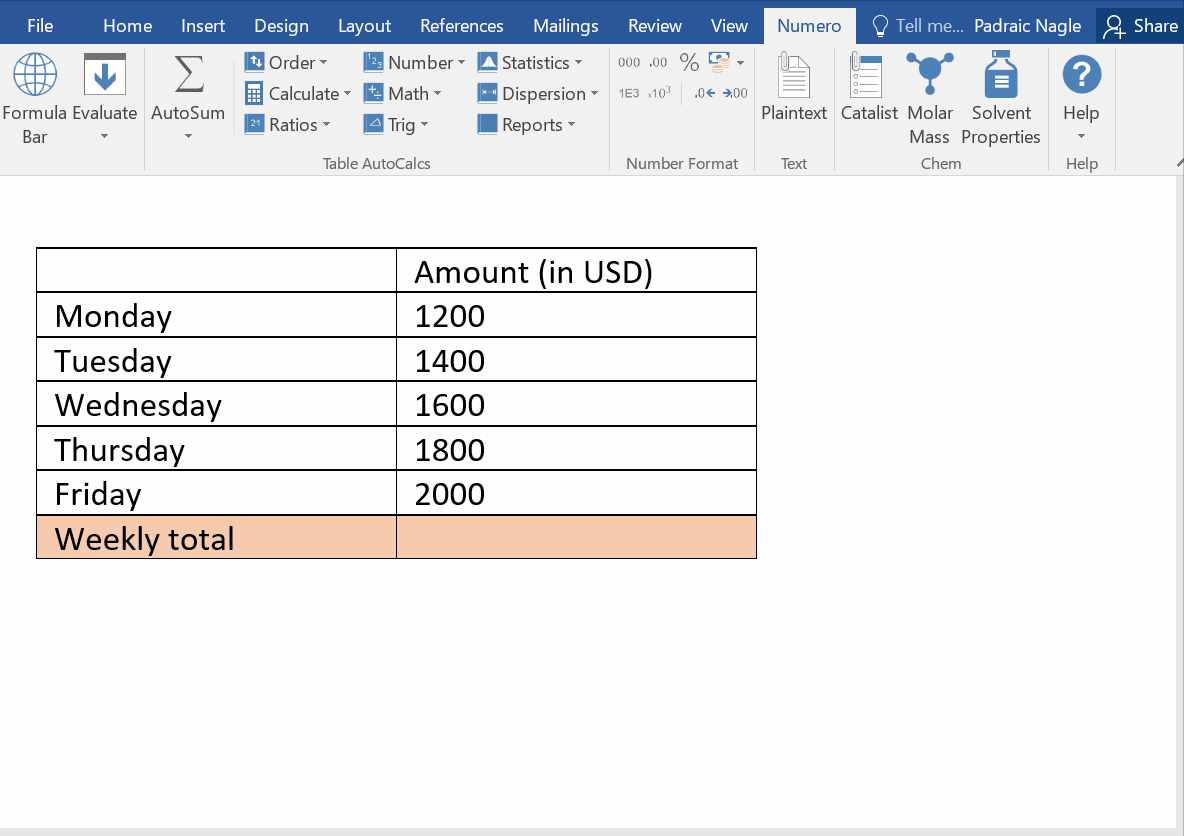2. Calculate the average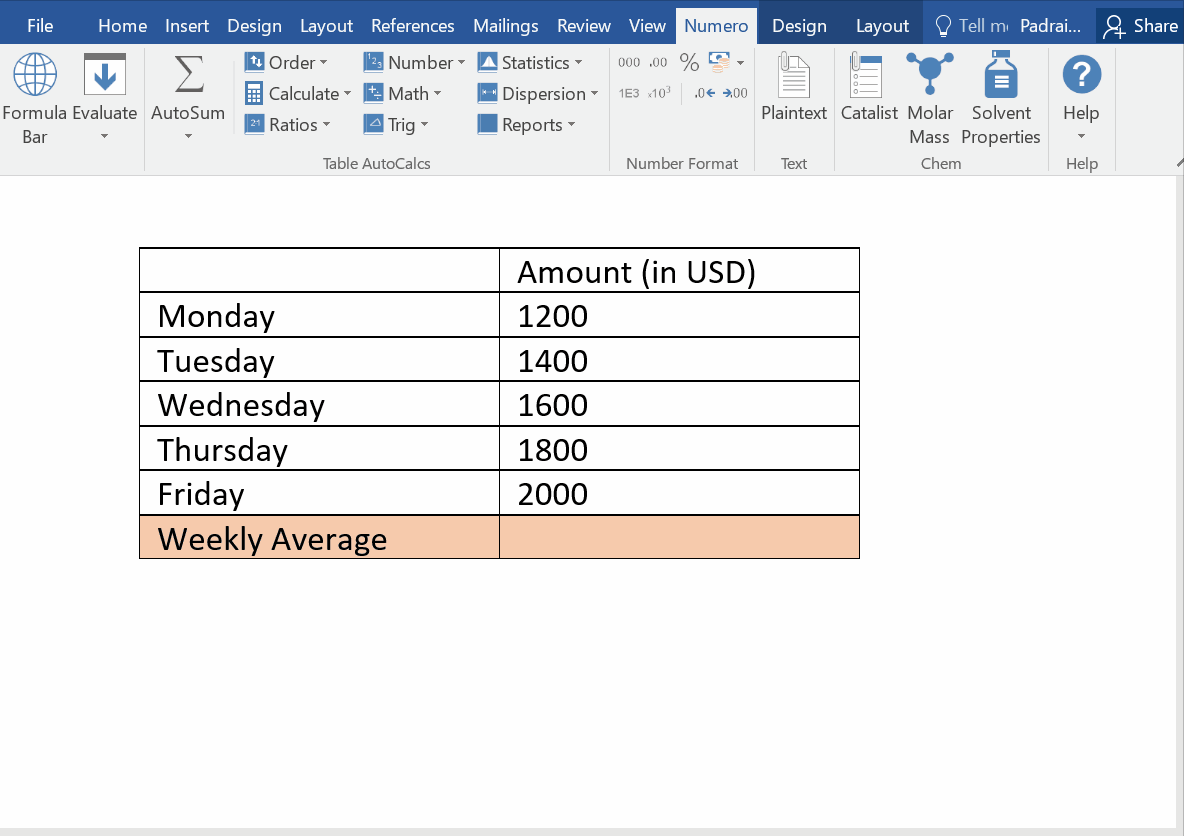3. Find the maximum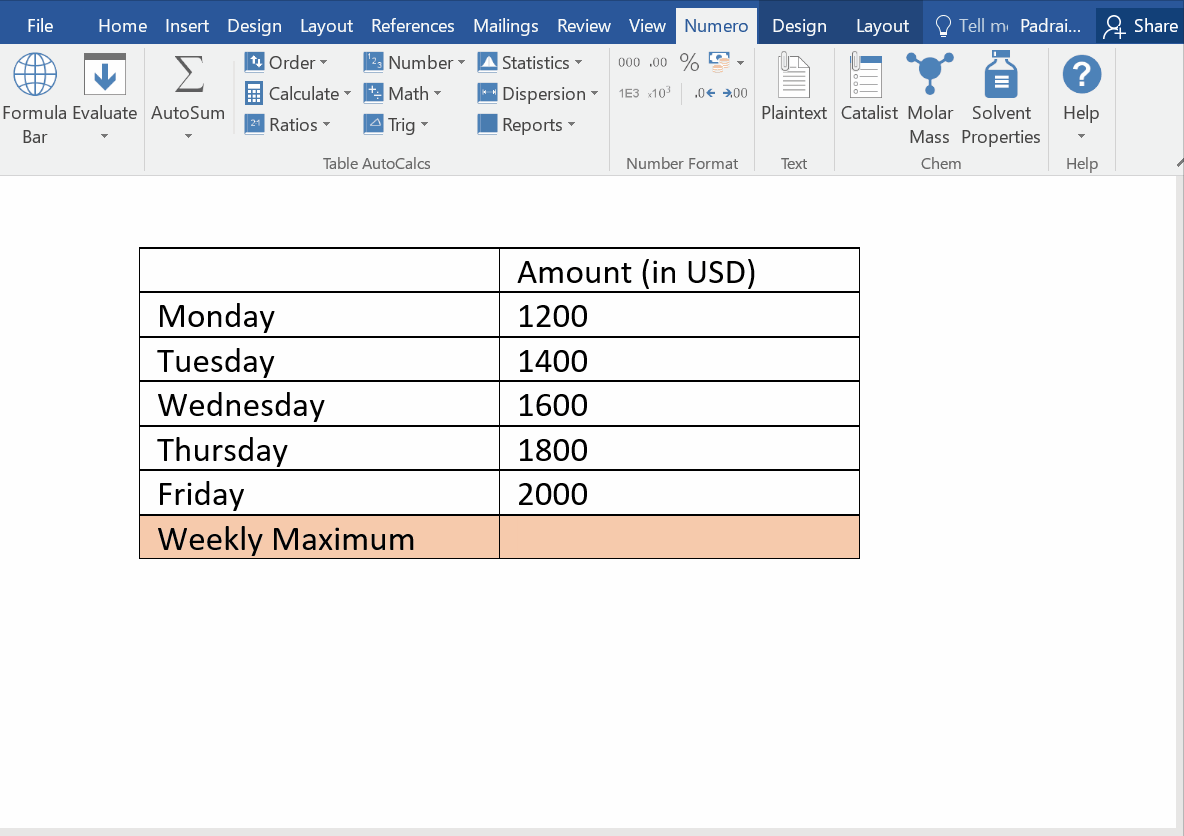AutoCalc descriptions

There are nine further subsections for table calculation enabling you to carry out simple calculations such as getting the sine, cosine or tangent of an array, to evaluating the geomean, harmean or the variance. These functions are divided into nine subsections, shown below: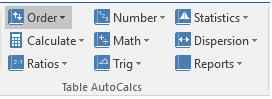The table below contains details of the functions in each subsection. Information on the function can be found here.

 Subsection Functions Order SORT(a), SORTD(a), REVERSE(a), RANDOMIZE(a), RANK(a;1), RANKAVG(a;1), NORMR(a), NORM(a) Calculate SUML(a), AVGL(a), MINL(a), MAXL(a), DIFF(a), DIFFN(a), DIFFF(a), DIFFL(a), SUMD(a), AVGD(a), MIND(a), MAXD(a) Ratio SUMX(a), SUMP(a), SUMLX(a), SUMLP(a), SUMDX(a), SUMDP(a), DIFFSUMX(a), DIFFSUMP(a) Number ROUND(a;0), ROUNDUP(a;0), ROUNDDOWN(a;0), TRUNC(a;0), ABS(a), NEG(a), INT(a) Math EXP(a), LOG(a), LN(a), LOG2(a), SQRT(a), CBRT(a), PRODUCT(a), FACT(a) Trig RADIANS(a), DEGREES(a), SIN(a), COS(a), TAN(a), COSEC(a), SEC(a), COTAN(a), ARCSIN(a), ARCCOS(a), ARCTAN(a), SIND(a), COSD(a), TAND(a), COSECD(a), SECD(a), COTAND(a), ARCIND(a), ARCCOSD(a), ARCTAND(a) Statistics MEDIAN(a), MEAN(a), MODMEAN(a), IQM(a), GEOMEAN(a), HARMEAN(a), QUADMEAN(a), CUBMEAN(a), VARP(a), VARS(a), SDEVP(a), SDEVS(a), SUMABS(a), SUMSQ(a), DEVSQ(a), ABSDEV(a), AVEDEV(a), MAD(a) Dispersion RANGE(a), MIDRANGE(a), Q1(a), Q2(a), Q3(a), IQR(a), FENCEL(a), FENCEU(a), OUTLIERS(a), BOXMIN(a), BOXMAX(a), MIDHINGE(a), TRIMEAN(a), D1(a), D9(a) Reports FREQUENCY(a), REP(a), REPFIVE(a), REPSEVEN(a), REPBOWLEY(a), REPSTATP(a), REPSTATS(a), REPBOX(a)

Examples:

1. Sort the following array of numbers in order from smallest to largest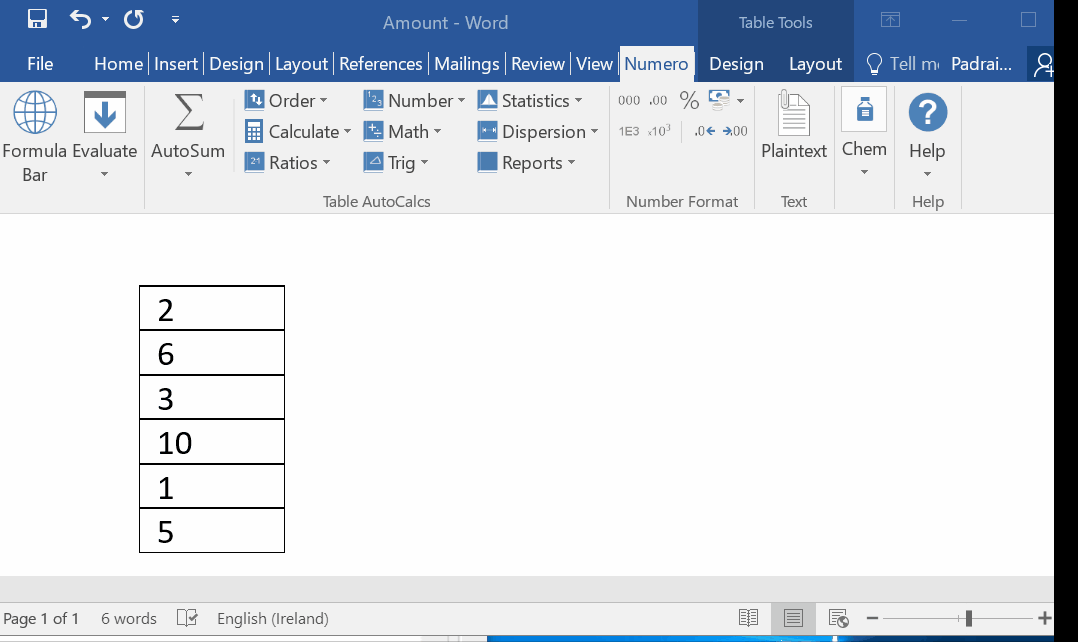2. Round the following array of numbesr to zero decimal places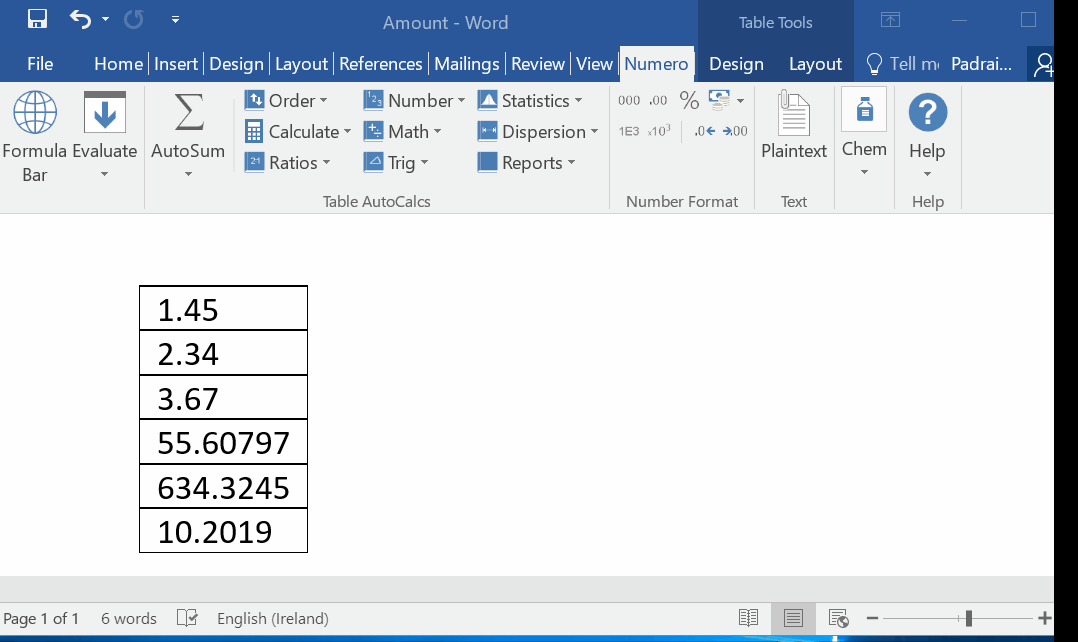3. Return the natural logarithm for the following array of numbers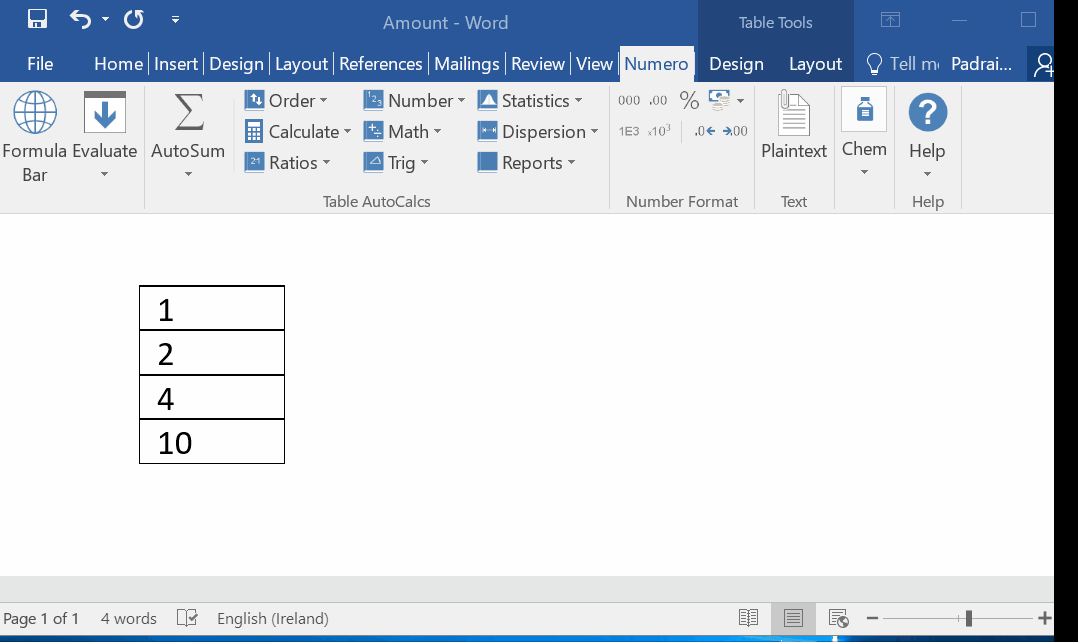4. Return the arithmetic mean for the following array of numbers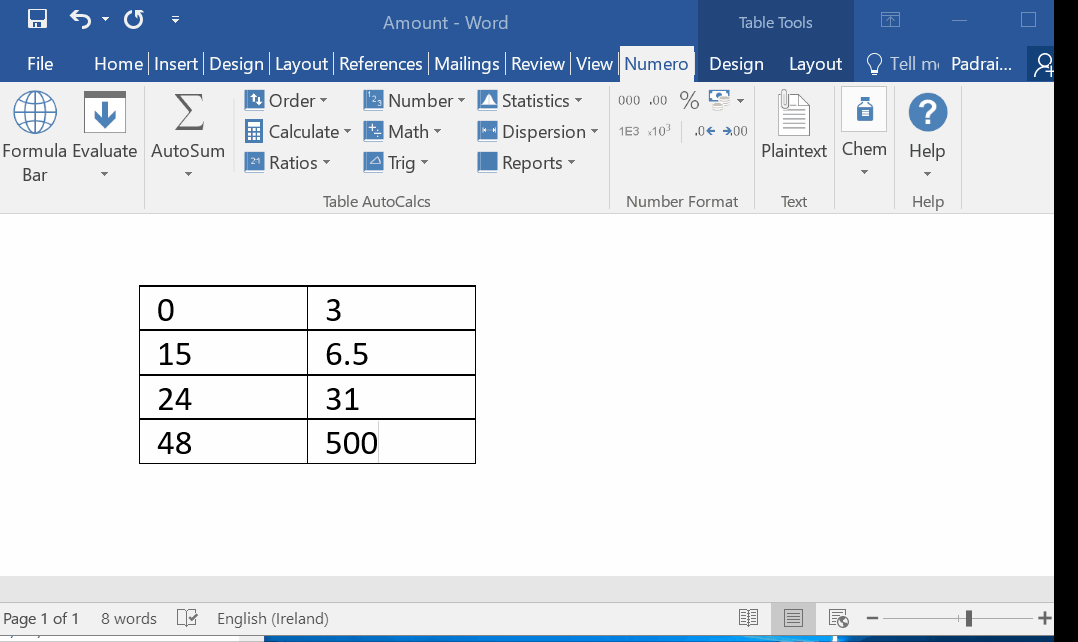6. Return the results for the functions:  SUM, AVERAGE, COUNT, MAX, MIN